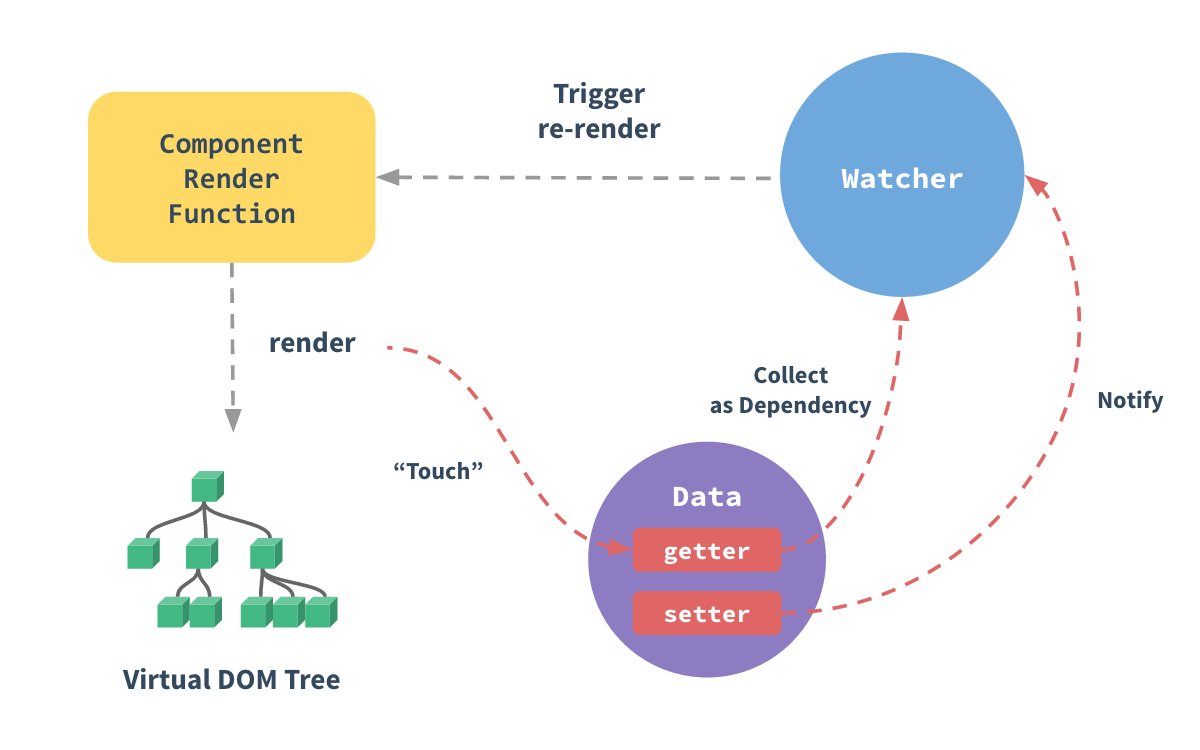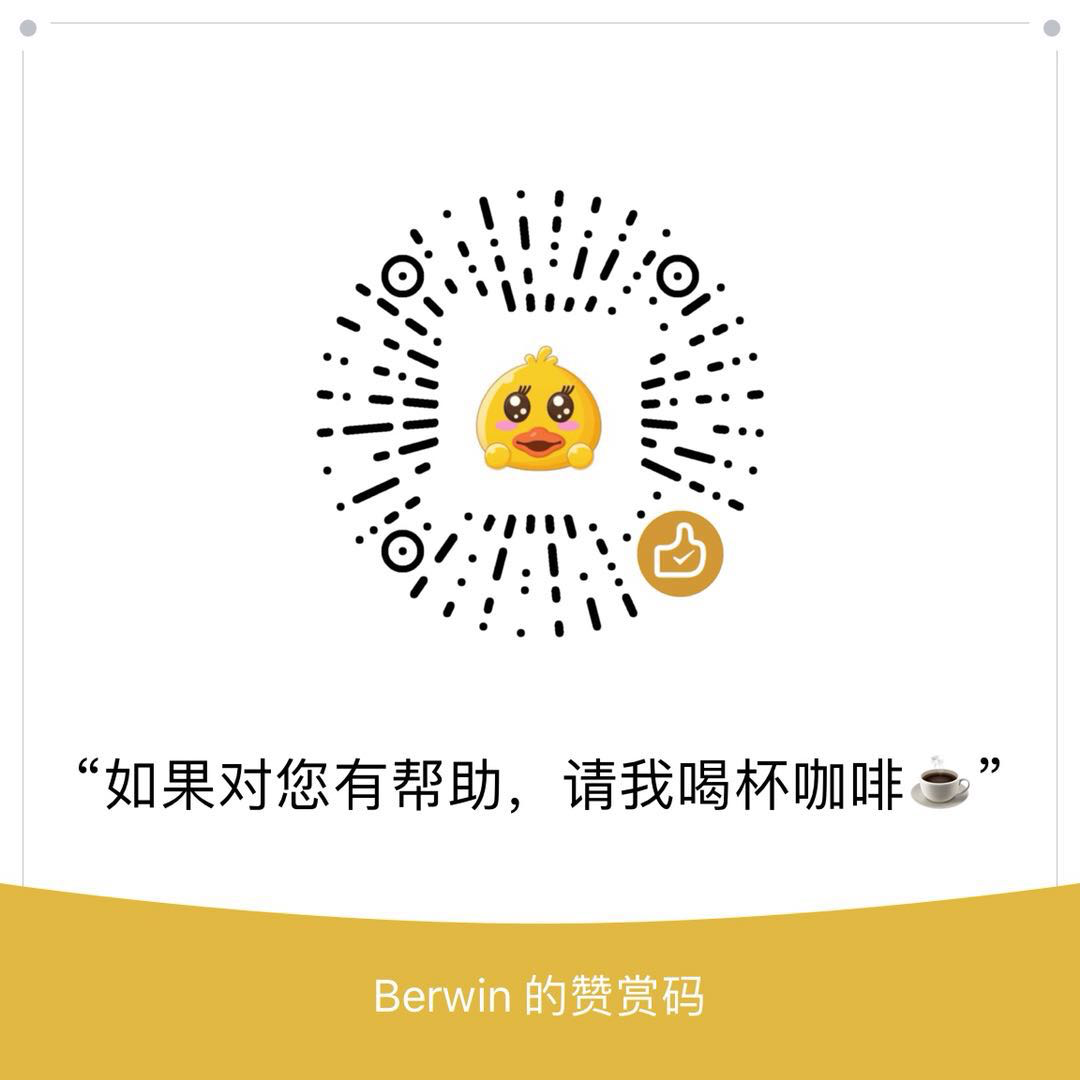# 深入浅出 - vue变化侦测原理

@berwin 2017-11-29 09:09:02发表于 berwin/Blog

# 深入浅出 - vue变化侦测原理

## 如何侦测变化？

```function defineReactive (data, key, val) {
Object.defineProperty(data, key, {
enumerable: true,
configurable: true,
get: function () {
return val
},
set: function (newVal) {
if(val === newVal){
return
}
val = newVal
}
})
}```

## 怎么观察？

```<template>
<div>{{ key }}</div>
<p>{{ key }}</p>
</template>```

## 依赖收集在哪？

```function defineReactive (data, key, val) {
let dep = [] // 新增
Object.defineProperty(data, key, {
enumerable: true,
configurable: true,
get: function () {
dep.push(window.target) // 新增
return val
},
set: function (newVal) {
if(val === newVal){
return
}

// 新增
for (let i = 0; i < dep.length; i++) {
dep[i](newVal, val)
}
val = newVal
}
})
}```

`defineReactive` 中新增了数组 dep，用来存储被收集的依赖。

```export default class Dep {
static target: ?Watcher;
id: number;
subs: Array<Watcher>;

constructor () {
this.id = uid++
this.subs = []
}

addSub (sub: Watcher) {
this.subs.push(sub)
}

removeSub (sub: Watcher) {
remove(this.subs, sub)
}

depend () {
if (Dep.target) {
this.addSub(Dep.target)
}
}

notify () {
// stabilize the subscriber list first
const subs = this.subs.slice()
for (let i = 0, l = subs.length; i < l; i++) {
subs[i].update()
}
}
}```

```function defineReactive (data, key, val) {
let dep = new Dep()        // 修改
Object.defineProperty(data, key, {
enumerable: true,
configurable: true,
get: function () {
dep.depend() // 修改
return val
},
set: function (newVal) {
if(val === newVal){
return
}

dep.notify() // 新增
val = newVal
}
})
}```

## 收集谁？## 什么是Watcher？

watcher 是一个中介的角色，数据发生变化通知给 watcher，然后watcher在通知给其他地方。

```// keypath
vm.\$watch('a.b.c', function (newVal, oldVal) {
// do something
})```

```class Watch {
constructor (expOrFn, cb) {
// 执行 this.getter() 就可以拿到 data.a.b.c
this.getter = parsePath(expOrFn)
this.cb = cb
this.value = this.get()
}

get () {
Dep.target = this
value = this.getter.call(vm, vm)
Dep.target = undefined
}

update () {
const oldValue = this.value
this.value = this.get()
this.cb.call(this.vm, this.value, oldValue)
}
}```

`update` 方法会触发参数中的回调函数，将value 和 oldValue 传到参数中。

## 递归侦测所有key

```// 新增
function walk (obj: Object) {
const keys = Object.keys(obj)
for (let i = 0; i < keys.length; i++) {
defineReactive(obj, keys[i], obj[keys[i]])
}
}

function defineReactive (data, key, val) {
walk(val) // 新增
let dep = new Dep()
Object.defineProperty(data, key, {
enumerable: true,
configurable: true,
get: function () {
dep.depend()
return val
},
set: function (newVal) {
if(val === newVal){
return
}

dep.notify()
val = newVal
}
})
}```

## Array怎么进行变化侦测？

vue 中对这个数组问题的解决方案非常的简单粗暴，我说说vue是如何实现的，大体上分三步：

vue的实现

```const arrayProto = Array.prototype
export const arrayMethods = Object.create(arrayProto)```

```;[
'push',
'pop',
'shift',
'unshift',
'splice',
'sort',
'reverse'
]
.forEach(function (method) {
// cache original method
const original = arrayProto[method]

Object.defineProperty(arrayMethods, method, {
value: function mutator (...args) {
console.log(method) // 打印数组方法
return original.apply(this, args)
},
enumerable: false,
writable: true,
configurable: true
})
})```

```// 工具函数
function def (obj: Object, key: string, val: any, enumerable?: boolean) {
Object.defineProperty(obj, key, {
value: val,
enumerable: !!enumerable,
writable: true,
configurable: true
})
}

export class Observer {
value: any;
dep: Dep;
vmCount: number; // number of vms that has this object as root \$data

constructor (value: any) {
this.value = value
this.dep = new Dep() // 新增
this.vmCount = 0
def(value, '__ob__', this) // 新增

// 新增
if (Array.isArray(value)) {
this.observeArray(value)
} else {
this.walk(value)
}
}

/**
* Walk through each property and convert them into
* getter/setters. This method should only be called when
* value type is Object.
*/
walk (obj: Object) {
const keys = Object.keys(obj)
for (let i = 0; i < keys.length; i++) {
defineReactive(obj, keys[i], obj[keys[i]])
}
}

/**
* Observe a list of Array items.
*/
observeArray (items: Array<any>) {
for (let i = 0, l = items.length; i < l; i++) {
new Observer(items[i])
}
}
}```

```;[
'push',
'pop',
'shift',
'unshift',
'splice',
'sort',
'reverse'
]
.forEach(function (method) {
// cache original method
const original = arrayProto[method]
def(arrayMethods, method, function mutator (...args) {
const result = original.apply(this, args)
const ob = this.__ob__
let inserted
switch (method) {
case 'push':
case 'unshift':
inserted = args
break
case 'splice':
inserted = args.slice(2)
break
}
if (inserted) ob.observeArray(inserted)
// notify change
ob.dep.notify()
return result
})
})```

## arrayMethods 是怎么生效的？

```export class Observer {
constructor (value: any) {
this.value = value
this.dep = new Dep()
this.vmCount = 0
def(value, '__ob__', this)

if (Array.isArray(value)) {
value.__proto__ = arrayMethods // 新增
this.observeArray(value)
} else {
this.walk(value)
}
}
}```

```// can we use __proto__?
const hasProto = '__proto__' in {} // 新增
export class Observer {
constructor (value: any) {
this.value = value
this.dep = new Dep()
this.vmCount = 0
def(value, '__ob__', this)

if (Array.isArray(value)) {
// 修改
const augment = hasProto
? protoAugment
: copyAugment
augment(value, arrayMethods, arrayKeys)
this.observeArray(value)
} else {
this.walk(value)
}
}
}

function protoAugment (target, src: Object, keys: any) {
target.__proto__ = src
}

function copyAugment (target: Object, src: Object, keys: Array<string>) {
for (let i = 0, l = keys.length; i < l; i++) {
const key = keys[i]
def(target, key, src[key])
}
}```

## 关于Array的问题

`this.list = 2`

`this.list.length = 0`

## 总结`getter``watcher` 有一条线，上面写着收集依赖，意思是说 `getter` 里收集 `watcher`，也就是说当数据发生 `get` 动作时开始收集 `watcher`

`setter``watcher` 有一条线，写着 `Notify` 意思是说在 `setter` 中触发消息，也就是当数据发生 `set` 动作时，通知 `watcher`

`Watcher` 到 ComponentRenderFunction 有一条线，写着 `Trigger re-render` 意思很明显了。